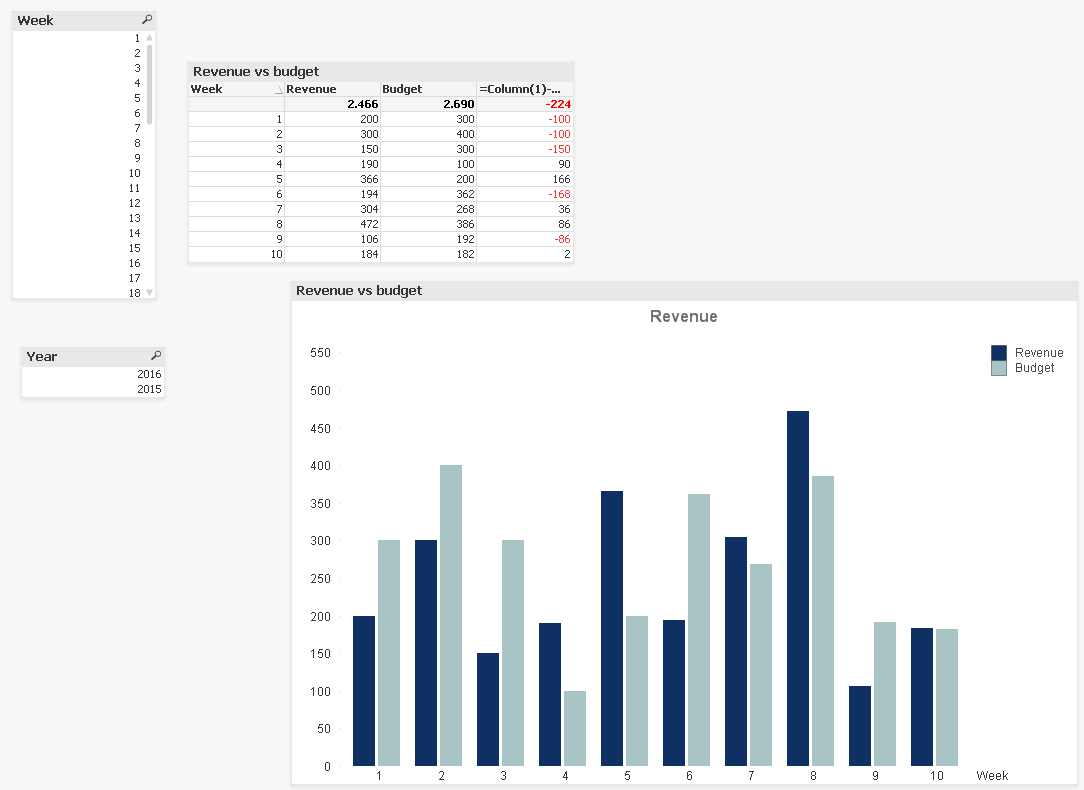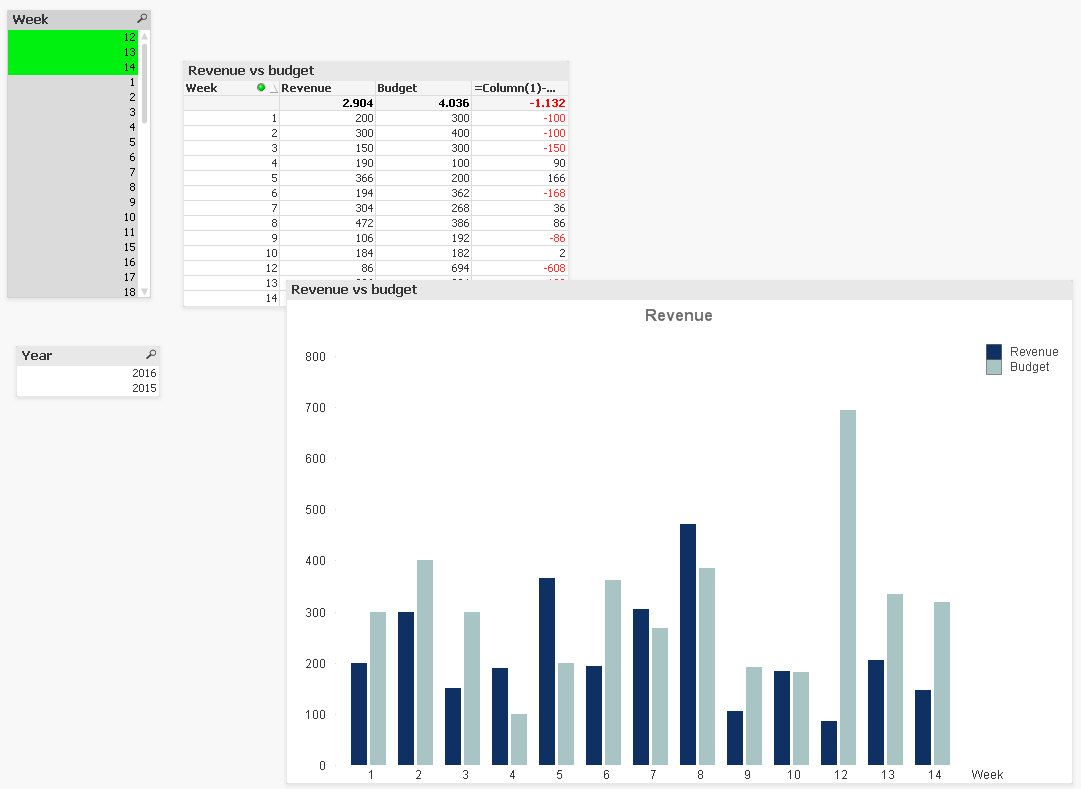# QlikView App Dev

Discussion Board for collaboration related to QlikView App Development.

cancel
Showing results for
Did you mean:Contributor III

## Set Analysis for certain Point in Time with a twist

Hi, Qlikview community

I have a problem I need to solve.

In my dataset I have Actual revenues and budget revenues and my users want to compare these number.

But I want to show only the current year and weeks that has passed when they open the tab.

So today is Year 2016 and week 10 then I want the user to only see that data in tables and charts. (see the qlikview file)

But sometimes the user want to see the budget for the next weeks e.g. budget for weeks 11,12,13.

So I want the options for the user to choose weeks 11, 12 and 13 and the user can see the budget for those weeks.

I use this formula to filter the data for max(year): Year ={\$(=max(Year))}   (see the qlikview file)

I used this formula for the budget to get only the budget fro the weeks that has passed: Date={'>=\$(=WeekStart(Today(), -53))<=\$(=Today())'}    (see the qlikview file)

But when I use these formulas I can't choose a week that has not passed e.g. 11,12, 13. I know the reason for that?

What can I do to change that? Do I need to change the formulas or can I do something?

regards Darri

1 Solution

Accepted SolutionsMVP

Like this?

No selectionWith selectionExpression for Actual Revenue

=If(GetSelectedCount(Week) = 0,

SUM({\$<Year ={\$(=max({<Week>}Year))}, Date ={'>=\$(=WeekStart(Today(), -53))<=\$(=Today())'}>}[Actual Revenue]),

SUM({\$+<Year ={\$(=max({<Week>}Year))}, Date ={'>=\$(=WeekStart(Today(), -53))<=\$(=Today())'}>}[Actual Revenue]))

Expression for Actual Budget

=If(GetSelectedCount(Week) = 0,

SUM({\$<Year ={\$(=max({<Week>}Year))}, Date ={'>=\$(=WeekStart(Today(), -53))<=\$(=Today())'}>}[Actual Budget]),

SUM({\$+<Year ={\$(=max({<Week>}Year))}, Date ={'>=\$(=WeekStart(Today(), -53))<=\$(=Today())'}>}[Actual Budget]))

5 RepliesMVP

Instead of \$(=Today()) use something like \$(=MakeWeekDate(max(Year),max(MyWeekNumber),6)) and let the user select the MyWeekNumber of their choice.

talk is cheap, supply exceeds demandMVP

May be like this:Expression for Actual Revenue

=If(GetSelectedCount(Week) = 0,

SUM({\$<Year ={\$(=max({<Week>}Year))}, Date ={'>=\$(=WeekStart(Today(), -53))<=\$(=Today())'}>}[Actual Revenue]),

SUM({\$+1<Year ={\$(=max({<Week>}Year))}, Date ={'>=\$(=WeekStart(Today(), -53))<=\$(=Today())'}>}[Actual Revenue]))

Expression for Actual Budget

=If(GetSelectedCount(Week) = 0,

SUM({\$<Year ={\$(=max({<Week>}Year))}, Date ={'>=\$(=WeekStart(Today(), -53))<=\$(=Today())'}>}[Actual Budget]),

SUM({\$+1<Year ={\$(=max({<Week>}Year))}, Date ={'>=\$(=WeekStart(Today(), -53))<=\$(=Today())'}>}[Actual Budget]))Contributor III
Author

Hi, Gysbert

with this formula I can choose week 11,12 and 13 which is what I want.

But when the user opens the tab the table shows every week and not only first 10 which I don't want.Contributor III
Author

Hi, Sunny

this solution is close to what I am thinking.

But when I choose for example week 11,12 and 13 I get all weeks from 1-13.

But I only want the weeks the user chooses.

But if the user does not choose anything is shows only week 1-10MVP

Like this?

No selectionWith selectionExpression for Actual Revenue

=If(GetSelectedCount(Week) = 0,

SUM({\$<Year ={\$(=max({<Week>}Year))}, Date ={'>=\$(=WeekStart(Today(), -53))<=\$(=Today())'}>}[Actual Revenue]),

SUM({\$+<Year ={\$(=max({<Week>}Year))}, Date ={'>=\$(=WeekStart(Today(), -53))<=\$(=Today())'}>}[Actual Revenue]))

Expression for Actual Budget

=If(GetSelectedCount(Week) = 0,

SUM({\$<Year ={\$(=max({<Week>}Year))}, Date ={'>=\$(=WeekStart(Today(), -53))<=\$(=Today())'}>}[Actual Budget]),

SUM({\$+<Year ={\$(=max({<Week>}Year))}, Date ={'>=\$(=WeekStart(Today(), -53))<=\$(=Today())'}>}[Actual Budget]))